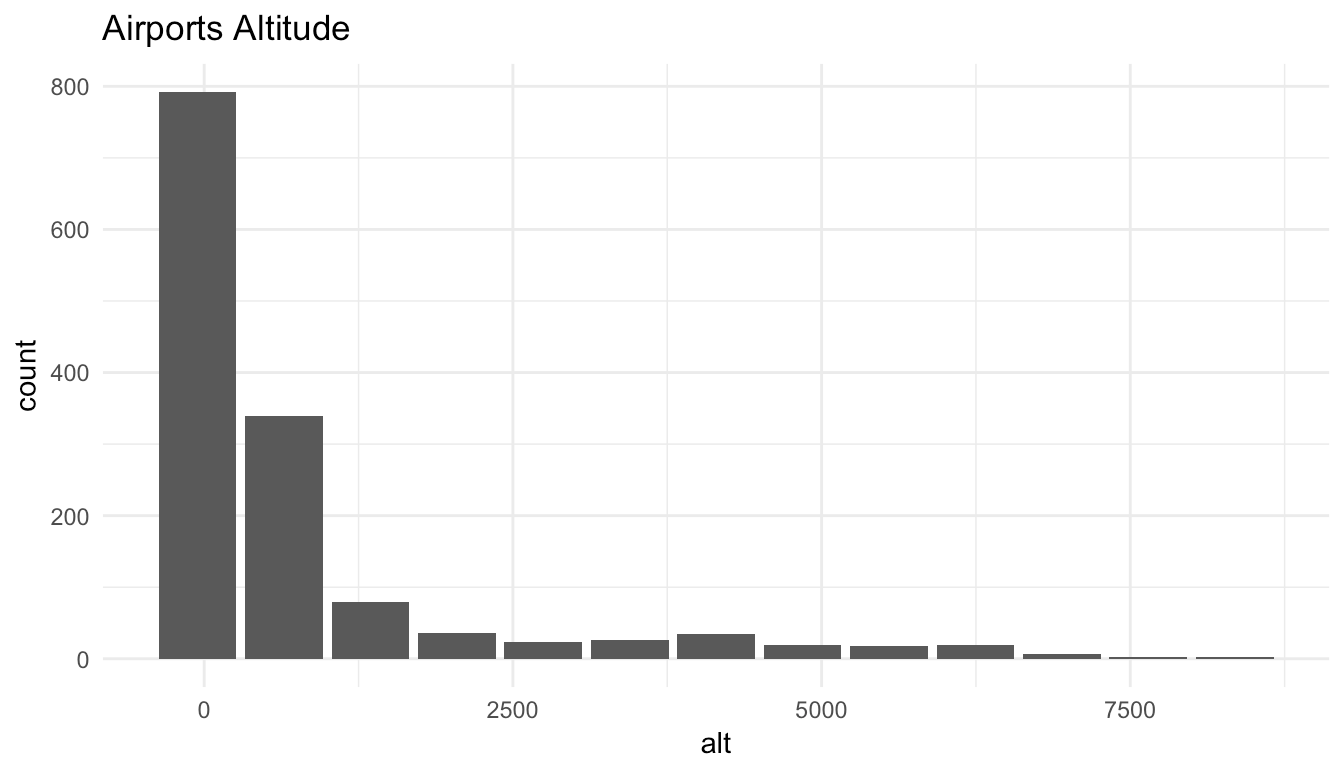Want to share your content on R-bloggers? click here if you have a blog, or here if you don't.

## The Challenge

Visualizations are one of R’s strengths. There are many functions and packages that create complex plots, often with one simple command. These plotting functions do two things: first, they take the raw data and run the calculations needed for a given visualization, and second, they draw the plot. If the source of the data resides within a database, the usual approach is to import all of the data and then create the plot. This is a problem, especially if the data is large.

A strategy to address this problem is found in the new Database with RStudio website. The Creating Visualizations page outlines a solution that introduces the “Transform in Database, plot in R” concept, and demonstrates its practical implementation. The article focused on knowledge sharing, rather than on providing a tool.

## Introducing `dbplot`

The new `dbplot` package is meant to collect multiple functions for in-database visualization code. It implements the principles laid out in the Creating Visualizations page, and it provides three types of functions:

• Helper functions that return a `ggplot2` visualization

• Helper functions that return the results of the plot’s calculations

• The `db_bin()` function introduced in the Creating Visualizations page

The package provides calculations or “base” `ggplot2` visualizations for the following:

• Bar plot

• Line plot

• Histogram

• Raster

## Installation

Install `dbplot` from GitHub using the `devtools` package

`devtools::install_github("edgararuiz/dbplot")`

## Example

This example will use a Microsoft SQL Server database connection to provide a quick glance of how the package works. For more examples, please visit the package’s GitHub repository.

### dbplot functions

The `dbplot_histogram()` function creates a 30-bin histogram by default. Because it uses `dplyr` commands to perform the bin calculations, the function will work with any database that has `dplyr` support, including `sparklyr`. The only caveat is that the database must support basic functions like `max()` and `min()`, which some database types do not support.

```library(dbplyr)

tbl(con, "airports") %>%
dbplot_histogram(alt)```This example shows how the resulting plot object can be further refined after the `dbplot_histogram()` function returns a plot:

```tbl(con, "airports") %>%
dbplot_histogram(alt, binwidth = 700) +
labs(title = "Airports Altitude") +
theme_minimal()```### db_compute functions

If more control over the plot is needed, then the `db_compute_bins()` function returns a data frame with the lowest value of each bin and the record count per bin:

```tbl(con, "airports") %>%
db_compute_bins(alt)```

```## # A tibble: 28 x 2
##       alt count
##     <dbl> <int>
##  1  -54.0   559
##  2  250.4   176
##  3  554.8   203
##  4  859.2   131
##  5 1163.6    82
##  6 1468.0    40
##  7 1772.4    20
##  8 2076.8    18
##  9 2381.2    16
## 10 2685.6    12
## # ... with 18 more rows```

The results of the compute command can then be piped into a plot:

```tbl(con, "airports") %>%
db_compute_bins(alt) %>%
ggplot() +
geom_col(aes(alt, count, fill = count))```### db_bin()

The `dbplot` package includes the `db_bin()` function, first introduced in the Creating Visualizations page. For more information, please read the Histogram section.

`db_bin(any_field)`

```## (((max(any_field) - min(any_field))/(30)) * ifelse((as.integer(floor(((any_field) -
##     min(any_field))/((max(any_field) - min(any_field))/(30))))) ==
##     (30), (as.integer(floor(((any_field) - min(any_field))/((max(any_field) -
##     min(any_field))/(30))))) - 1, (as.integer(floor(((any_field) -
##     min(any_field))/((max(any_field) - min(any_field))/(30))))))) +
##     min(any_field)```

## Next steps

More plots will be possible as `dplyr`-to-SQL translations are fine-tuned and enhanced. The `dbplot` package will be the place where new calculations and plots will be implemented.